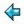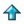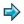### genmat.m

The matrix package again, but parameterised over an arbitrary element type, with a zero, a unit and four functions of arithmetic. Example - to instantiate this package with numbers as the element type, in another script, say:-
```    %include <ex/genmat> { elem==num; zero=0; unit=1;
plus=+; minus=-; times=*; divide=/; }
```
However another possibility would be to use the package to do matrix calculations over rationals (as defined in <ex/rational>) thus:-
```    %include <ex/genmat>
{ elem==rational; zero=mkrat 0; unit=mkrat 1;
plus=rplus; minus=rminus; times=rtimes; divide=rdiv; }
```

```%export matrix idmat matadd matsub matmult prescalmult postscalmult

%free { elem :: type
zero, unit :: elem
plus, minus, times, divide :: elem->elem->elem
}

matrix == [[elem]]

idmat :: num->matrix ||identity matrix of given size
idmat n = [[delta i j|j<-[1..n]]|i<-[1..n]]
where
delta i j = unit, if i=j
= zero, otherwise

where
vadd x y = map2 plus x y

matsub :: matrix->matrix->matrix
matsub x y = map2 vsub x y
where
vsub = map2 minus

matmult :: matrix->matrix->matrix
matmult x y = outer inner x (transpose y)  ||*
inner x y = summate (map2 times x y)
outer f x y = [[f a b|b<-y]|a<-x]

||*note that transpose is already defined in the standard environment

summate = foldl plus zero

prescalmult :: elem->matrix->matrix ||premultiply a matrix by a scalar
prescalmult n x = map (map (times n)) x

postscalmult :: elem->matrix->matrix ||postmultiply a matrix by a scalar
postscalmult n x = map (map (\$times n)) x

||we need both the above because element multiplication may not be
||commutative

mkrow :: [elem]->matrix ||make vector into matrix with a single row
mkrow x = [x]

mkcol :: [elem]->matrix ||make vector into matrix with a single column
mkcol x = map (:[]) x

det :: matrix->elem ||determinant, of square matrix
det [[a]] = a
det xs = summate [(xs!0!i) \$times cofactor 0 i xs|i<-index xs], if #xs=#xs!0
= error "det of nonsquare matrix",                       otherwise
cofactor i j xs = parity (i+j) \$times det (minor i j xs)
minor i j xs = [omit j x | x<-omit i xs]
omit i x = take i x ++ drop (i+1) x

parity::num->elem
parity i = unit,             if i mod 2 = 0
= zero \$minus unit, otherwise